﻿ Kinematic Analysis of Planar Parallel MechanismPublications are Open
Access in this journal
Article Versions
Export Article
• Normal Style
• MLA Style
• APA Style
• Chicago Style
Research Article
Open Access Peer-reviewed

Kinematic Analysis of Planar Parallel Mechanism

František Trebuňa, Michal Kelemen, Ivan Virgala , Alexander Gmiterko
American Journal of Mechanical Engineering. 2017, 5(6), 303-306. DOI: 10.12691/ajme-5-6-14
Published online: December 15, 2017

Abstract

The paper deals with kinematic model of parallel mechanism equipped with elastic members. The main aim is to determine the workspace of particular point of the mechanism in order to designing of whole mechanism for the future. Whole mechanisms should consist of several same segments which creates concept of serial mechanism. Since there is investigated parallel mechanism, at first has to be solved inverse kinematic model in order to obtain generalized variables, which are necessary for direct kinematic model. In the paper Jacobian matrix is derived for investigated mechanism. Then the algorithm for inverse kinematic model is described. The inverse kinematic model uses damped least squares method which appears as suitable method for our purposes. The results of inverse kinematic solution is consequently used for direct kinematic model, which is described by homogeneous transformation matrices. The planar parallel mechanism is simulated in software Matlab and the results are expressed in the graphs as well as our approach is discussed in the conclusion.

1. Introduction

In the paper is investigated planar parallel mechanism, which is one part of whole positioning system consisting of the same parallel mechanisms (segments). These individual parallel segments (closed chains) forming one serial mechanism (open chain). The system is analyzed and designed for positioning of cryomagnets for Compact Linear Collider.

The precise positioning of cryomagnets is realized by complex of robotic modules with extremely high precision. One segment of mentioned serial mechanism is considered as planar parallel kinematic mechanism allowing motions in junction plane (the plane, where next positioning mechanism – segment is connected into concept, so-called snake-like positioning system), see Figure 1.

• Figure 1. Preliminary design of positioning system

Blue color represents linear actuators by which the one segment is positioned. Simplified model of designed system in the Figure 2 is shown.

• Figure 2. Simplified model of investigated system

The designed positioning system is composed of many same segments. In this work will be considered only vertical linear actuators. Under the actuators are placed the elastic members in order to change the properties of designed mechanism. Deformation of these members is conditioned by applied forces on mechanism and therefore they will be considered only as dependent generalized variables within the kinematic model.

2. Kinematic Analysis of Simplified Model

The kinematic analysis deals with simplification of model, presented in the introduction. There are several approaches for investigation of kinematic model of parallel mechanism. Our approach is based on replacing parallel mechanism with serial mechanism and solving at first the inverse kinematics instead of direct kinematics. 1

Simplified model of one segment in the Figure 3 is shown.

• Figure 3. Kinematic scheme of simplified model of investigated planar parallel segment

In the Figure 3, the red color shows elastic members which will be represented by dependent generalized variables. Black color shows the construction of investigated planar parallel segment. In the points A and B are placed linear actuators which are represented by independent generalized variables. As can be seen from this figure, there is parallel (closed chain) mechanism which is fixed with ground in two points (A, B). The variables q1 and q5 represents linear actuators by which whole construction can moves and be positioned according to requirements.

The aim of this study is to investigate the workplace of control point E. By knowing of workspace of point E can be evaluated weather the range of linear actuators motion is suitable.

The vector of generalized variables is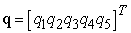(1)

where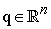and n is DOF (degrees of freedom) of mechanism. To find the workspace of control point E can be applied following view. The mechanism is fixed with ground in the point A and the point B is float point. By this consideration can be whole mechanism investigated as mechanism with serial kinematic structure, see Figure 4.

• Figure 4. Different view on investigated mechanism – serial kinematic structure

Mentioned simplification changes the situation and the task of direct kinematic model is now divided into two tasks. Now, the task is to find such configuration of generalized variables, in which the point B will have position of fixation with ground, according to initial position in the Figure 3. The point B has to be in the position [d,0], see Figure 5. 5

• Figure 5. Simplified model for inverse kinematic task

In other words, at first the inverse kinematic solution have to be find and then can be investigated the workspace of point E by direct kinematic model.

3. Algorithm for Finding of Workspace of Parallel Mechanism

One of the key elements within tasks of kinematics and dynamics of industrial robots and manipulators is Jacobian matrix J. Using numerical approach for solving introduced problem is necessary to described Jacobian matrix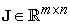, where m represents dimension of main task (what in the case of planar task is 2) and n is DOF of mechanism.

The position of the point B relative to point A is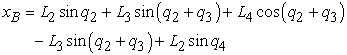(2)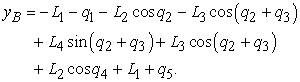(3)

Particular elements of Jacobian matrix are based on derivation of functions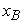and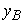according to particular generalized variables of vector q.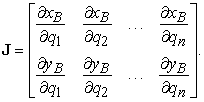(4)

It is usually very difficult to find analytical solution for inverse kinematic tasks, in most cases it is impossible, especially for kinematically redundant mechanisms. From this reason is often used numerical approach, based on 2, 4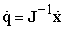(5)

where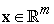. The equation (5) is solved by iteration cycle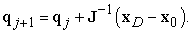(6)

The equation (6) can be used for non-real-time applications. Vector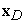is vector of required positions of end-effector (point B) and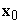is vector of initial values of point B. Since, Jacobian matrix is not square matrix but matrix with dimension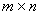, there has to be used any method for Jacobian matrix modification like pseudoinverse of Jacobian matrix (Moore-Penrose) or its transposition, etc. In our case will be used damped least squares method based on following relation 3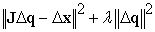(7)

where λ represents nonzero constant. By means of mentioned method can be solved problems around singular states of mechanism. Final solution is given by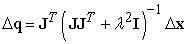(8)

where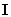is unit matrix. The solution of inverse kinematic task can be expressed by following algorithm.

Algorithm 1: Inverse kinematic solution

1: CYCLE FOR 1

2: Definition of new generalized variable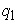3: CYCLE FOR 2

4: Definition of new generalized variable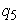5: CYCLE FOR 3

6: Definition of new generalized variable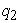7: CYCLE WHILE 1

8: Calculation of Jacobian matrix J

9: Determination of point B position considering updated variables10: Calculation of generalized equation11: Rewriting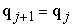12: Determination, if calculated position of point B is identical with required position (considering of tolerance) If Yes – END OF CYCLE WHILE 1 If No – continue of CYCLE WHILE 1

13: END of CYCLE WHILE 1

14: Drawing of mechanism position

15: END of CYCLE FOR 3

16: END of CYCLE FOR 2

17: END of CYCLE FOR 1

By above mentioned algorithm one can obtain the vector of generalized variables q. Cycles FOR 1 and FOR 2 ensure that variablesandchange from the minimum to maximum extension of linear actuators. Variablerepresents elastic member of mechanism, other generalized variables which represent elastic members of the mechanism are calculated in CYCLE WHILE 1. By FEM analysis can be determined maximum value of elastic members deformation and based on this can be stated admissible value of variables,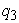and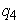.

The result of each configuration of generalized variables vector q is consequently used for direct kinematic solution in order to determine workspace of control point E.

For direct kinematic model the homogeneous transformation matrices are used. The global coordinate system (GCS) is placed in the point A. Control point E is now like end-effector, whose position is investigated. In the Figure 6, the particular local coordinate systems are shown and position of control point E is investigated related to the GCS.

• Figure 6. Model for direct kinematic solution

The transformations from GCS to particular local coordinate systems (LCS) can be expressed by following equations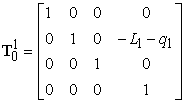(9)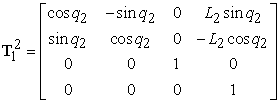(10)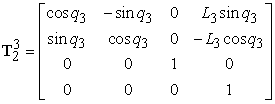(11)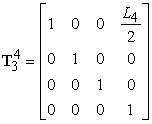(12)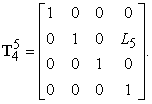(13)

The equation (9) represents the transformation of the first linear actuator, equations (10) and (11) express deformations in elastic member as function ofand. The equations (12) and (13) express transformations from elastic member to control point E.

The final relation between control point E and GCS can be written as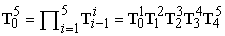(14)

Using the equation (14) can be determined the workspace of control point E.

4. Simulation of Kinematic Model

The simulation of inverse and direct kinematic solution of investigated parallel mechanism was done in software Matlab. For simulation were used following parameters of mechanism: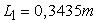,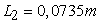,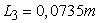,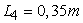,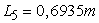,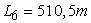,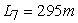and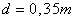. Calculating tolerance for inverse kinematic solution was 0,0005 m. The inverse kinematic solution uses damped least squares method with scalar variable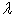= 1,2. Range of motion of linear actuators is ± 0,001 m. Step of actuators position during the simulation is 0,0005 m.

Motion of mechanism during the simulation can be seen in the Figure 7.

• Figure 7. Simulation of motion of parallel mechanism

Black color and red color represent construction of the mechanism and elastic members, respectively. As have been mentioned, the results of inverse kinematic solution were used as input to direct kinematic model. Then the workspace of control point E can be determined.

The workspace of the point E in the Figure 8 is shown.

• Figure 8. Workspace of control point E

Of course, the lower the step of actuator position is (in the simulation), the more precise the determination of workspace would be. Analysis and investigation of workspace of point E can be consequently used for analysis and design of whole system, which will consists of many the same segments.

5. Conclusion

The one segment of positioning snake-like mechanism is investigated considering planar model. The aim of the paper is to determine workspace of parallel mechanism moving by two linear actuators. The simplified model of mechanism has 5 DOF. For determination of control point workspace at first was derived inverse kinematic model. Inverse kinematic model uses tolerance 0,0005 m. By numeric simulation the vector of generalized variables is calculated. This vector is consequently used for direct kinematic model and by homogeneous transformation matrices can be determined the workspace of control point. These results can be used for the future for designing of whole positioning system.

Acknowledgements

This paper was supported by project Stimuli No. Req-00169-0003 Research and development of intelligent mobile robotic platforms and positioning systems with high precision for using in research, development and industry.

References

  S. R. Buss, Introduction to Inverse Kinematics with Jacobian Transpose, Pseudoinverse and Damped Least Squares methods, IEEE Journal of Robotics and Automation (2004), pp. 681-685. In article View Article  D. Koniar, L. Hargaš, M. Hrianka, V. Bobek, P. Drgoňa, P. Fibich, Kinematics analysis of biomechanical systems using image analysis, Metalurgija (Metallurgy), Vol. 49 (2/2010), ISSN 1334-2576. In article  H. Shen, H. Wu, B. Chen, Y. Jiang, C. Yan, Obstacle Avoidance Algorithm for 7-DOF Redundant Anthropomorphic Arm, Journal of Control Science and Engineering, Hindawi Publishing Corporation 2015. In article View Article  P. H. Chang, Analysis and Control of Robot Manipulators with Kinematic Redundancy, PhD Thesis MIT 1987. In article View Article  F. Aghili, Adaptive Control of Manipulators Forming Closed Kinematic Chain With Inaccurate Kinematic Model, IEEE/ASME Transactions on Mechatronics, Volume: 18, Issue: 5, 2013. In article View Article

Published with license by Science and Education Publishing, Copyright © 2017 František Trebuňa, Michal Kelemen, Ivan Virgala and Alexander GmiterkoThis work is licensed under a Creative Commons Attribution 4.0 International License. To view a copy of this license, visit http://creativecommons.org/licenses/by/4.0/